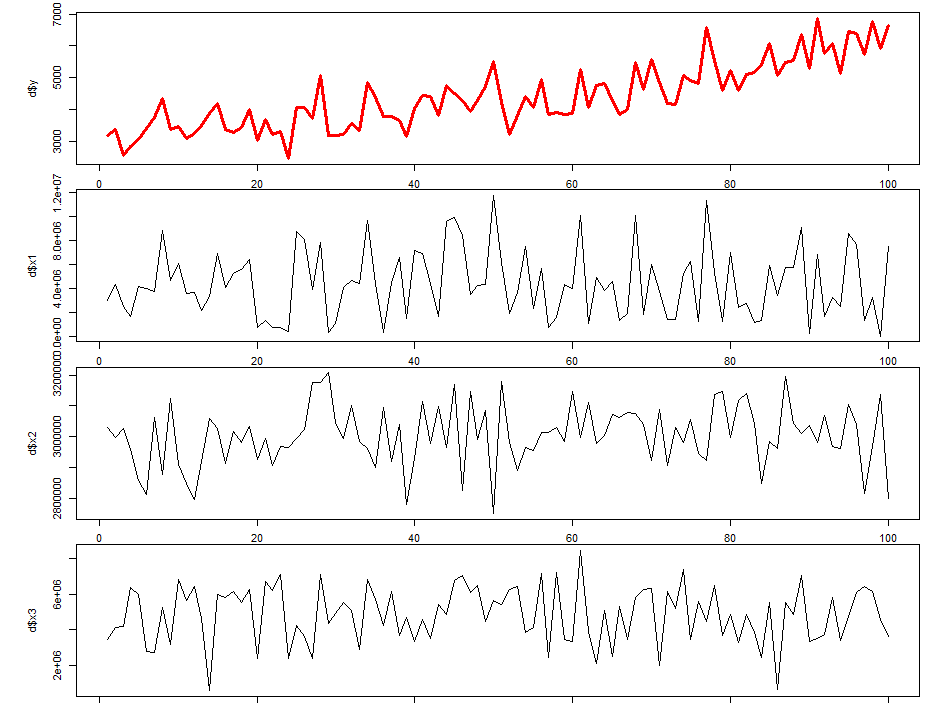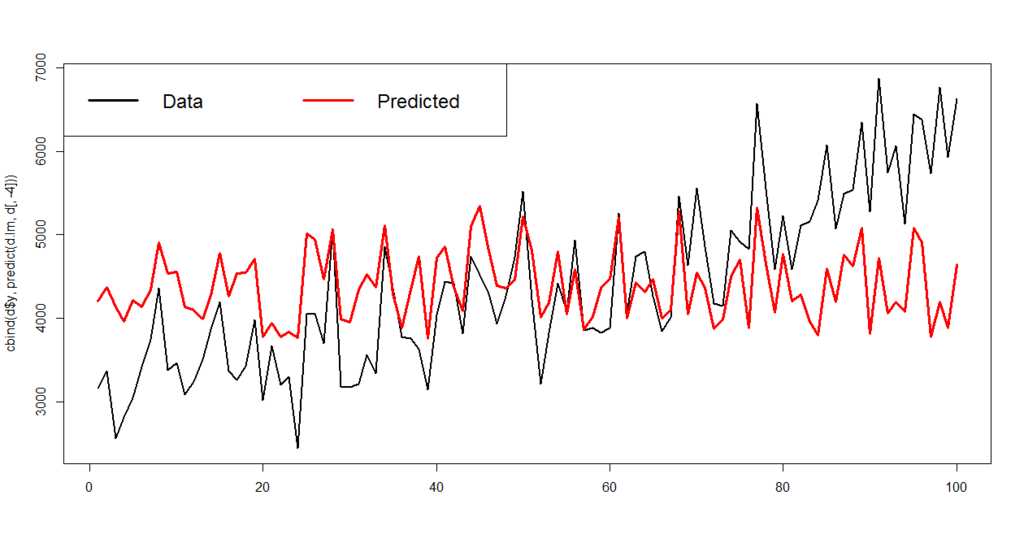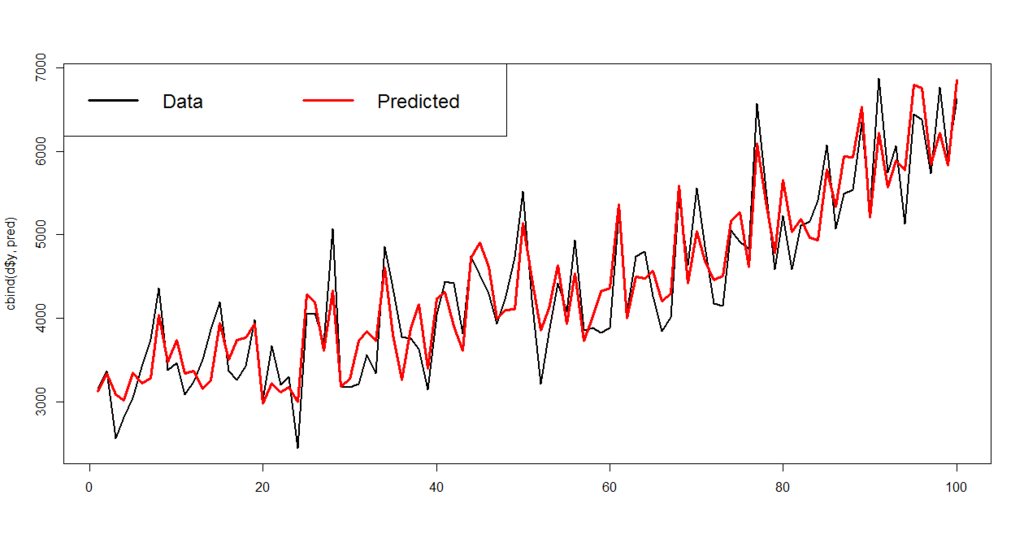# Bayesian modeling with R and Stan (4): Time series with a nonlinear trend

The previous post reviewed how to estimate a simple hierarchical Bayesian models. You can see more complicated cases in a great textbook "The BUGS book".

But personally hierarchical Bayesian modeling is the most useful for time-series analysis. I think {dlm} CRAN package is popular for such a purpose, but in order to run more complicated modeling Stan would be a powerful alternative.

To see a simple practice on a complicated time series analysis with Stan, first download a sample dataset from GitHub and import it as "d" to your RStudio workspace. It contains 3 independent variables (x1, x2 and x3) and 1 dependent variable (y). Here I assume y means a certain the number of conversion on a daily basis and x1-x3 mean daily amounts of budget for distinct ads. You can overview how they are just by plotting.

> par(mfrow=c(4,1),mar=c(1,6,1,1))
> plot(d$y,type='l',lwd=3,col='red') > plot(d$x1,type='l',lwd=1.5)
> plot(d$x2,type='l',lwd=1.5) > plot(d$x3,type='l',lwd=1.5)It appears to include some nonlinear trend. Usual multiple linear regression cannot fit such a time series.

> d.lm<-lm(y~.,d)
> matplot(cbind(d$y,predict(d.lm,d[,-4])),type='l',lty=1,lwd=3,col=c(1,2))In traditional econometrics, such a trend should be treated as, for example, unit root process or trend process. But here we tackle it with just a Bayesian modeling using Stan. First, let's fit it using a simple model with second-order difference trend. The model is described as follows.Actually I set parameters as a = 0.00015, b = 0.00025, c = 5e-05, d = 1000 in the sample dataset. OK, can we correctly estimate these parameters with ruling out a nonlinear trend? Below is a Stan code for the model above. Save it as "hb_ts1.stan". data { int<lower=0> N; real<lower=0> x1[N]; real<lower=0> x2[N]; real<lower=0> x3[N]; real<lower=0> y[N]; } parameters { real trend[N]; real s_trend; real s_q; real<lower=0> a; real<lower=0> b; real<lower=0> c; real d; } model { real q[N]; real cum_trend[N]; for (i in 3:N) trend[i]~normal(2*trend[i-1]-trend[i-2],s_trend); cum_trend<-trend; for (i in 2:N) cum_trend[i]<-cum_trend[i-1]+trend[i]; for (i in 1:N) q[i]<-y[i]-cum_trend[i]; for (i in 1:N) q[i]~normal(a*x1[i]+b*x2[i]+c*x3[i]+d,s_q); }  So now just run as below. > library(rstan) > dat<-list(N=100,x1=d$x1,x2=d$x2,x3=d$x3,y=d$y) > fit1<-stan(file='hb_ts1.stan',data=dat,iter=1000,chains=4) > library(coda) > fit1.coda<-mcmc.list(lapply(1:ncol(fit1),function(x) mcmc(as.array(fit1)[,x,]))) > plot(fit1.coda)  You can check its convergence here. Let's estimate each parameter. > fit1.smp<-extract(fit1) > dens_a<-density(fit1.smp$a)
> dens_b<-density(fit1.smp$b) > dens_c<-density(fit1.smp$c)
> dens_d<-density(fit1.smp$d) > a_est<-dens_a$x[dens_a$y==max(dens_a$y)]
> b_est<-dens_b$x[dens_b$y==max(dens_b$y)] > c_est<-dens_c$x[dens_c$y==max(dens_c$y)]
> d_est<-dens_d$x[dens_d$y==max(dens_d$y)] > trend_est<-rep(0,100) > for (i in 1:100) { + tmp<-density(fit1.smp$trend[,i])
+     trend_est[i]<-tmp$x[tmp$y==max(tmp$y)] + } > pred<-a_est*d$x1+b_est*d$x2+c_est*d$x3+d_est+cumsum(trend_est)
> par(mfrow=c(1,1))
> matplot(cbind(d\$y,pred),type='l',lty=1,lwd=c(2,3),col=c(1,2))
> legend('topleft',legend=c('Data','Predicted'),col=c(1,2),lty=1,lwd=3,ncol=2,cex=1.5)> a_est
 0.0001447182
> b_est
 0.0003036063
> c_est
 2.771731e-05
> d_est
 1669.245Great, it looks very nice! But actually a certain problem still remains to solve. In the next post, we'll try it.